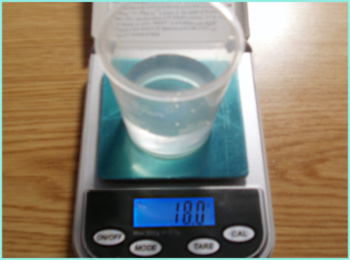Knowledge database: Stoichiometry: Molar mass Molar mass is the mass of an amount of substance that contains the Avogadro's number of particles, which is equal to 6.0221415x1023. In other words, the mass of the mentioned number of particles of a substance is the molar mass of that substance. Since the weight of different particles (atoms, molecules or ions) differs, each substance has an unique molar mass, although there are cases where two different molecules are composed of the same number of different atoms, but these atoms are arranged differently and are often connected with different chemical bonds and because of that, they actually form different compounds. Relative atomic mass that is shown in the periodic table of elements is numerically equal to the molar mass of the element that is observed. However, one should have in mind that in case of the molar mass, the unit which expresses the molar mass must be added - gram per mole (g/mol). In the case of molecules, the molar mass can be calculated by simply summing up the relative atomic masses of the atoms, i.e. elements that form the molecule, and adding the g/mol unit.In the case of water, there is one atom of oxygen (15,999) + two atoms of hydrogen (2 x 1.008), which gives 18,015 g/mol. On the picture above, one can see approximately one mole of water.